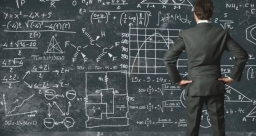# Eq1

When the product of two less than three times a number and four is subtracted from nine times the number, the result is eight more than five times the number.

What is the number?Did you find an error or inaccuracy? Feel free to write us. Thank you!Tips to related online calculators
Do you have a linear equation or system of equations and looking for its solution? Or do you have a quadratic equation?